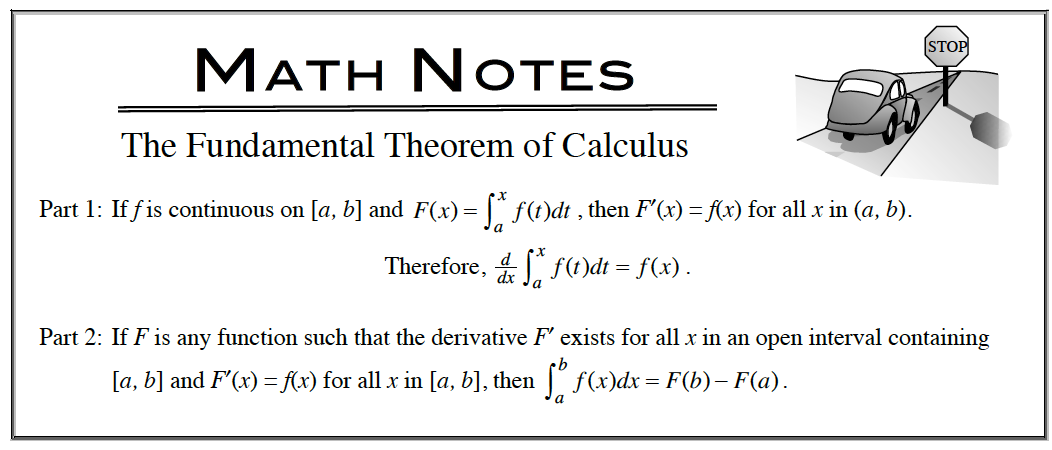### Home > APCALC > Chapter 6 > Lesson 6.4.2 > Problem6-135

6-135.

Without using a calculator, evaluate the following expressions. Homework Help ✎1. $\frac { d } { d x }( \int _ { 2 } ^ { x } \sqrt { 9 - x ^ { 3 } } d x )$1. $\frac { d } { d t }(t^{−1}\sin(t))$

Product Rule or Quotient Rule, depending how you write $t^{ −1}$.

1. $\int [ \frac { d } { d x } ( \frac { x + \operatorname { tan } ( x ) } { x - \operatorname { sec } ( x ) } + 5 ) ] d x$

Refer to the hint in part (a). But notice that this is an integral of a derivative, not the reverse. That means that this answer will include +C.

1. $\int _ { 0 } ^ { 1 } [ \frac { 1 } { \sqrt { 1 - x ^ { 2 } } } + \operatorname { sec } ^ { 2 } ( x ) ] d x$

Both parts of the sum in the integrand have antiderivatives that are trig (or inverse trig) functions.